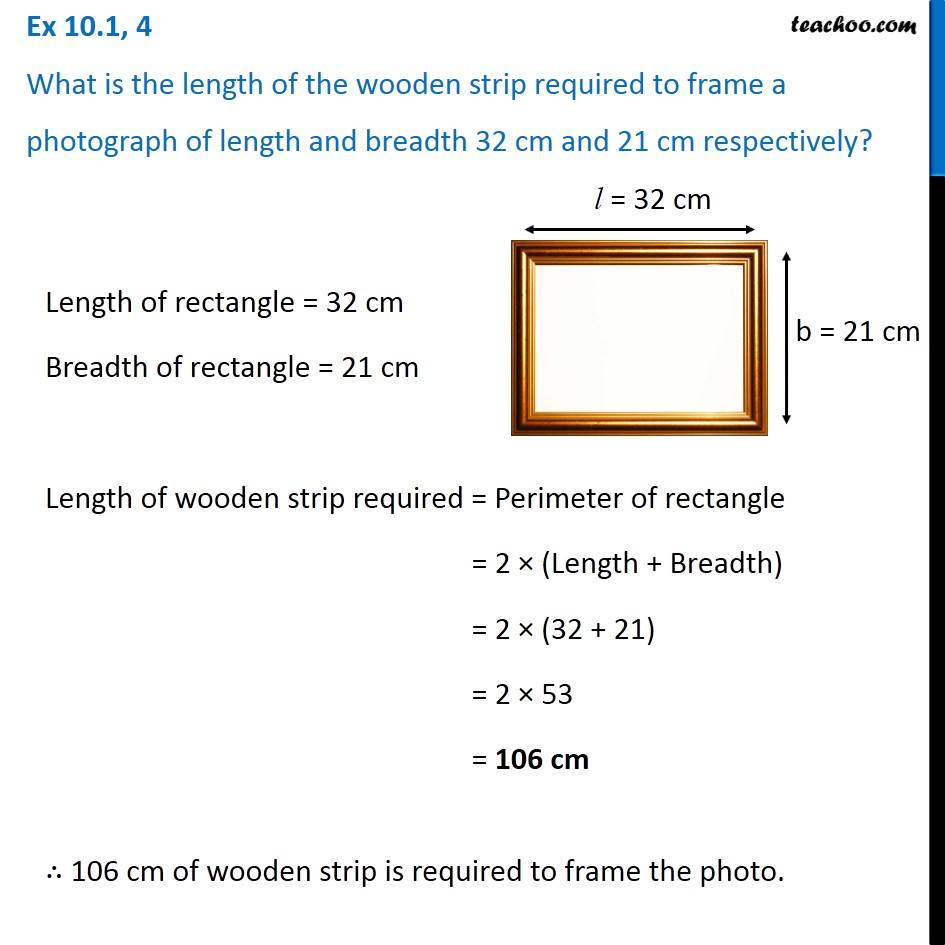Subscribe to our Youtube Channel - https://you.tube/teachoo

1. Chapter 10 Class 6 Mensuration
2. Concept wise
3. Perimeter of Rectangle

Transcript

Ex 10.1, 4 What is the length of the wooden strip required to frame a photograph of length and breadth 32 cm and 21 cm respectively? Length of rectangle = 32 cm Breadth of rectangle = 21 cm Length of wooden strip required = Perimeter of rectangle = 2 × (Length + Breadth) = 2 × (32 + 21) = 2 × 53 = 106 cm ∴ 106 cm of wooden strip is required to frame the photo.

Perimeter of Rectangle